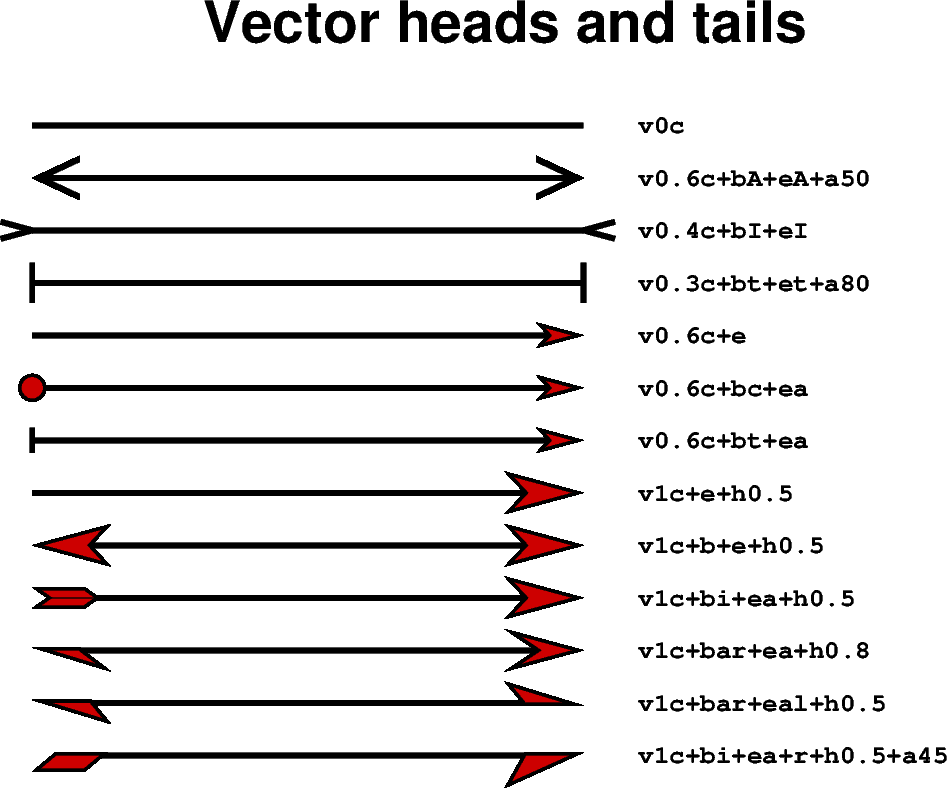Many methods in PyGMT allow plotting vectors with individual heads and tails. For this purpose, several modifiers may be appended to the corresponding vector-producing parameters for specifying the placement of vector heads and tails, their shapes, and the justification of the vector.

To place a vector head at the beginning of the vector path simply append +b to the vector-producing option (use +e to place one at the end). Optionally, append t for a terminal line, c for a circle, a for arrow (default), i for tail, A for plain open arrow, and I for plain open tail. Further append l or r (e.g. `+bar`) to only draw the left or right half-sides of the selected head/tail (default is both sides) or use +l or +r to apply simultaneously to both sides. In this context left and right refer to the side of the vector line when viewed from the beginning point to the end point of a line segment. The angle of the vector head apex can be set using +aangle (default is 30). The shape of the vector head can be adjusted using +hshape (e.g. `+h0.5`).

For further modifiers see the Vector Attributes subsection of the corresponding method.

In the following we use the `pygmt.Figure.plot` method to plot vectors with individual heads and tails. We must specify the modifiers (together with the vector type, here `v`, see also Vector types documentation) by passing the corresponding shortcuts to the `style` parameter.```<IPython.core.display.Image object>
```

```import pygmt

fig = pygmt.Figure()
fig.basemap(
region=[0, 10, 0, 15], projection="X15c/10c", frame="+tVector heads and tails"
)

x = 1
y = 14
angle = 0  # in degrees, measured counter-clockwise from horizontal
length = 7

for vecstyle in [
# vector without head and tail (line)
"v0c",
# plain open arrow at beginning and end, angle of the vector head apex is
# set to 50
"v0.6c+bA+eA+a50",
# plain open tail at beginning and end
"v0.4c+bI+eI",
# terminal line at beginning and end, angle of vector head apex is set
# to 80
"v0.3c+bt+et+a80",
"v0.6c+e",
# circle at beginning and arrow head at end
"v0.6c+bc+ea",
# terminal line at beginning and arrow head at end
"v0.6c+bt+ea",
# arrow head at end, shape of vector head is set to 0.5
"v1c+e+h0.5",
# modified arrow heads at beginning and end
"v1c+b+e+h0.5",
# tail at beginning and arrow with modified vector head at end
"v1c+bi+ea+h0.5",
# half-sided arrow head (right side) at beginning and arrow at the end
"v1c+bar+ea+h0.8",
# half-sided arrow heads at beginning (right side) and end (left side)
"v1c+bar+eal+h0.5",
# half-sided tail at beginning and arrow at end (right side for both)
"v1c+bi+ea+r+h0.5+a45",
]:
fig.plot(
x=x, y=y, style=vecstyle, direction=([angle], [length]), pen="2p", fill="red3"
)
fig.text(
x=6, y=y, text=vecstyle, font="Courier-Bold", justify="ML", offset="0.2c/0c"
)
y -= 1  # move the next vector down

fig.show()
```

Total running time of the script: ( 0 minutes 4.083 seconds)

Gallery generated by Sphinx-Gallery